# Harmonic polynomial

(diff) ← Older revision | Latest revision (diff) | Newer revision → (diff)

A polynomial withas variables that satisfies the Laplace equation. Any harmonic polynomial may be represented as the sum of homogeneous harmonic polynomials. If, there are only two linearly independent homogeneous harmonic polynomials of degree— for example, the real and the imaginary part of the expression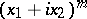. If, the number of linearly independent homogeneous polynomials of degreeis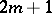. In the general case —— the number of linearly independent homogeneous harmonic polynomials of degreeiswhere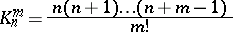is the number of permutations ofobjects takenat a time withrepetitions. The homogeneous harmonic polynomials,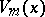, are also known as spherical functions (in particular if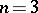). If, one may write, in spherical coordinateswhere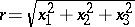and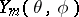is a spherical function of degree.

How to Cite This Entry:
Harmonic polynomial. Encyclopedia of Mathematics. URL: http://encyclopediaofmath.org/index.php?title=Harmonic_polynomial&oldid=16082
This article was adapted from an original article by V.F. Emel'yanov (originator), which appeared in Encyclopedia of Mathematics - ISBN 1402006098. See original article